Published: 28 November 2019

# Intelligent glove for suppression of resting tremor in Parkinson’s disease

Asan G. A. Muthalif2
Diyana N. H. Nordin3
Thaer M. I. Syam4
1, 3Smart Structures, Systems and Control Research Lab (S3CRL) Department of Mechatronics Engineering, Kulliyyah of Engineering, International Islamic University, Kuala Lumpur, Malaysia
2, 4Departament of Mechanical and Industrial Engineering, College of Engineering, Qatar University, Doha, Qatar
Corresponding Author:
Asan G. A. Muthalif
Views 746

#### Abstract

One of the significant symptoms in Parkinson’s disease is resting tremor. Resting tremor occurs when the muscle is relaxed, causing the limb to shake. Rhythmic muscle movement of the patients commonly happens within the range of 4 Hz to 6 Hz. Thus, reducing this type of tremor will help improve patients’ quality of life. In this paper, to suppress resting tremors, an intelligent glove was designed utilizing the concepts of vibrations and gyro effect. A rotating brass disc attached to the glove creates a gyroscopic effect of the smart glove. Therefore, the disc will do their utmost to stay upright and counter any input forces instantaneously by providing the counterforce. A reduction of more than 50 % with the intelligent glove is also shown.

#### Highlights

• The article recommends intelligent glove for suppression of vibrations for resting tremors of Parkinson’s disease.
• A prototype model is fabricated to validate performance of the glove.
• Experimental study on the prototype has shown up to 90 % reduction for fixed sine signal between 3 Hz-7 Hz.

## 1. Introduction

Parkinson’s disease is a neurodegenerative disorder that affects human limb movements . Dr. James Parkinson first discussed it in “An Essay on the Shaking Palsy”, which was published in 1817 . Primary symptoms of Parkinson’s disease include shaking and trembling. Parkinson’s disease tremor can range from 4-5 Hz for low frequency and 8-10 Hz for high frequency . Patients suffering from tremors might face difficulties in their daily activities as muscle movements are involuntary. There are two types of tremors, namely resting tremor (RT) and action tremor (AT) with resting tremor being recognized as the primary symptom of Parkinson’s disease [4, 5]. Resting tremor occurs at a frequency of 4-6 Hz when the limbs are at rest and disappears with voluntary movements [4, 6]. On the other hand, action tremor occurs during voluntary movements .

Currently, no cure has been found to treat Parkinson’s disease . Nevertheless, medical approaches such as medications and surgeries can slow down the progress rate towards disability. However, patients are prone to the side effects of these treatments, and the dosage of the medicine has to be gradually increased as the patients’ body get accustomed to the regular dosage .

Though several assistive devices have been designed for Parkinson’s disease patients, they are, however expensive and are usually function-oriented. For example, [8, 9] designed a spoon to assist patients for self-feeding. On the other hand, [10, 11] developed a mobility device to improve patients’ walking gait. Furthermore,  developed a monitoring system to use in a diverse Parkinson’s disease population for at least two weeks.  determined the influence of dopamine agonist treatment on the prevalence of Parkinson’s disease disturbances. This paper aims at eliminating vibrations so that the patients for daily activities need only one device. In this work, a wearable glove using gyroscopic technology is designed to suppress vibrations due to resting tremors experienced by the Parkinson’s patients.

## 2. Vibration suppression of rest tremor utilizing an intelligent glove

The intelligent glove designed for vibration suppression of patients’ hand consists of a gyroscope, a motor, an aluminium frame as well as a Velcro hook and loop fastening strap. It utilizes gyroscopic force, which works based on the principle of angular momentum. The spinning discs located inside the gyroscope counters any input force instantaneously. Fig. 1(a) shows the front view of the intelligent glove, which is modelled in a spring-mass mechanism as in Fig. 1(b).

Fig. 1The intelligent glove, worn on a mannequin hand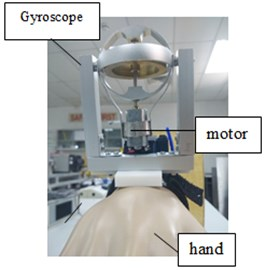a)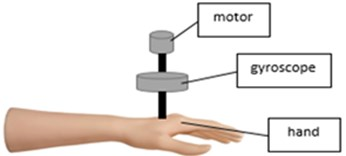b)

### 2.1. Mathematical modelling of the intelligent glove

The wheel of the gyroscope can be used to derive the mathematical modelling of the gyroscope, which is attached to the intelligent glove. Deriving mathematical modelling of a system is important to know the behaviour of the system.

Fig. 2Reference diagram for the wheel analysis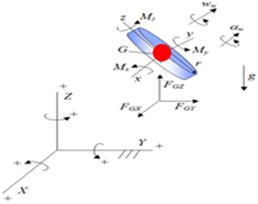Based on the local $xyz$ axis as well as using trigonometry’s equations, the summation of moments about point $G$ as in, $\sum {M}_{G}$ of the gyroscope wheel is written as :

1
$\sum {M}_{G}^{x}={I}_{G}^{x}{\alpha }^{x}-\left({I}_{G}^{y}-{I}_{G}^{z}\right){\omega }^{y}{\omega }^{z},$
2
$\sum {M}_{G}^{y}={I}_{G}^{y}{\alpha }^{y}-\left({I}_{G}^{z}-{I}_{G}^{x}\right){\omega }^{z}{\omega }^{x},$
3
$\sum {M}_{G}^{z}={I}_{G}^{z}{\alpha }^{z}-\left({I}_{G}^{x}-{I}_{G}^{y}\right){\omega }^{x}{\omega }^{y},$

where $I$ is the mass moment of inertia, $\alpha$ is the angular acceleration and $\omega$ represents angular velocity. However, no tangential acceleration exists since point $G$ is travelling in a horizontal circle, resulting in ${\alpha }^{y}={\alpha }^{z}=0$. Thus, substituting this information into Eqs. (2, 3) will make ${M}_{G}^{y}={M}_{G}^{z}=0$. Also, the mass moment of inertia about point $G$, in $x$, $y$ and $z$ directions can be expressed as:

4
${I}_{G}^{x}={I}_{G}^{z}=\frac{1}{4}{m}_{w}{r}^{2},$
5
${I}_{G}^{y}=\frac{1}{2}{m}_{w}{r}^{2},$

where $r$ and $m$ is the radius and mass of the wheel, respectively. In terms of angular velocity, the angular acceleration term in $x$-axis can be expressed as:

6
${\alpha }^{x}=-{\omega }_{s}{\omega }_{p}\mathrm{s}\mathrm{i}\mathrm{n}\theta .$

Here, subscripts $s$ and $p$ refer to the spin rate of the wheel and precession of the wheel. Thus, substituting Eq. (4-6) into Eq. (1), the equation can finally be written as in Eq. (7). This expression represents the necessary amount of moment needed to counter the resting force from the patients’ hand:

7
${M}_{G}^{x}=-\frac{1}{4}{m}_{w}{r}^{2}{\omega }_{s}{\omega }_{p}\mathrm{s}\mathrm{i}\mathrm{n}\theta -\frac{1}{4}{m}_{w}{r}^{2}\left({\omega }_{s}+{\omega }_{p}cos\theta \right){\omega }_{p}\mathrm{s}\mathrm{i}\mathrm{n}\theta .$

## 3. Experimental studies of the intelligent glove

### 3.1. Experimental setup

The experimental setup, as shown in Fig. 3, consists of HP 3570A dynamic signal analyser (DSA), an accelerometer, a power amplifier, an inertial shaker and a mannequin hand wearing the glove. The accelerometer was used to capture the signals and was directly connected to the dynamic system analyser so that the output can be analysed and saved. The input excitations were also provided by the DSA, which was fed to the inertial shaker through the power amplifier to mimic resting tremor. In this work, the result is displayed in the form of a transfer function, which is the ratio of the accelerometer readings to the input voltage (g/V). Experiments were conducted with and without the intelligent glove to study the performance of the proposed device, in two modes, i.e. swept sine and fixed sine.

Fig. 3Experimental setup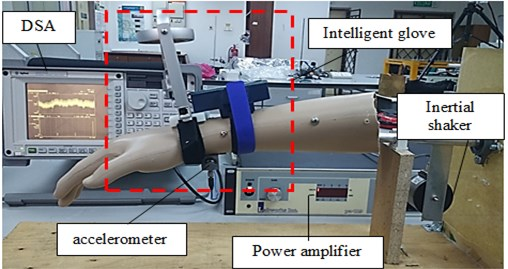It is assumed that the highest frequency of the resting tremor is at 7 Hz. The parameters used in designing the gyroscope are listed in Table 1.

Table 1Parameters of the gyroscope and the mannequin hand

 Parameter Value Mass of mannequin hand 1.064 kg Length of mannequin hand 0.8 m Mass of gyroscope 0.145 kg

### 3.2.1. Performance of the intelligent glove subjected to swept sine signal

The experiment was initially fed with a swept sine signal within the range of Parkinson’s disease frequency, which is 4 Hz $ 7 Hz. In this range, as seen from Fig. 4, a huge difference between the uncontrolled and controlled system can be observed. Using the intelligent glove, a reduction of more than 50 % of the acceleration can be seen.

Fig. 4Controlled and uncontrolled performances, when swept from 4 Hz to 7 Hz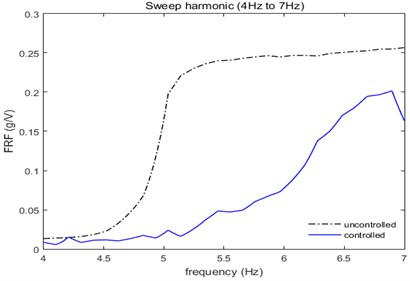### 3.2.2. Performance of the intelligent glove subjected to fixed sine signal

In this section, a fixed harmonic signal (at 4 Hz, 5 Hz, 6 Hz and 7 Hz, respectively) was applied to the system. The performance of the system, with and without the intelligent glove, was studied.

The performance of the intelligent glove in term of normalized frequency response function (FRF) at 4 Hz is shown in Fig. 5(a). At the frequency of 4 Hz, a reduction of about 50 % with the intelligent glove is seen. Fig. 5(b) shows the plots when subjected to 5 Hz of fixed sine signal. The mannequin hand experienced 6.8 times of reduction in vibrations when wearing the intelligent glove.

Fig. 5Controlled and uncontrolled performances at a) 4 Hz and b) 5 Hz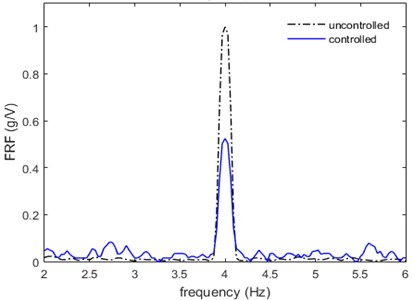a)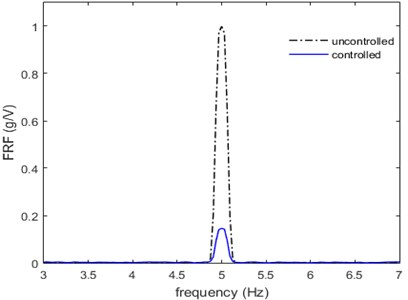b)

At 6 Hz as it can be observed in Fig. 6(a) without the glove, the hand experienced 18.8 mg/V of acceleration. However, the intelligent glove managed to suppress vibrations to 2.8 mg/V. Thus, a reduction of 85 % is seen on the graph. Finally, in Fig. 6(b), about 90 % of vibration reduction is seen for 7 Hz.

Fig. 6Controlled and uncontrolled performances at a) 6 Hz and b) 7 Hz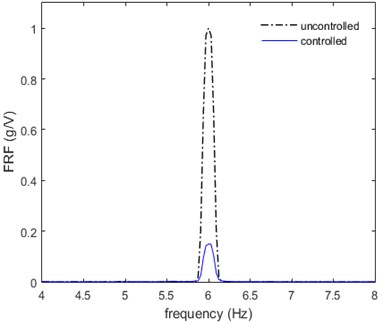a)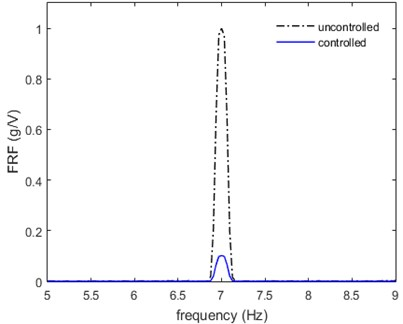b)

From the results presented in this section and by looking at the normalized FRF by which the ratio of output acceleration/input voltage is plotted against the frequency, it can be seen that the proposed intelligent glove can cause a significant reduction in the acceleration/voltage amplitude so that it can be used to reduce vibrations due to resting tremors, as experienced by Parkinson’s disease patients. A minimum of 50 % vibration suppression was recorded during the experiment.

## 4. Conclusions

The intelligent glove is appropriate for reduction of vibrations due to resting tremors for Parkinson’s disease patients that occurs at a frequency of 3 Hz-7 Hz. Results showed that a reduction of more than 50 % for a swept sine excitation. Also, it showed a minimum of 50 % and a maximum of 90 % reduction in for a fixed sine signal at all frequencies ranges between 3 Hz-7 Hz. The proposed glove is suitable for suppression of vibrations for resting tremors of Parkinson’s disease, and it only uses one device instead of using several devices so that patients can be more comfortable.

#### Cited by

Recent Trends in Mechatronics Towards Industry 4.0
4D printing of soft orthoses for tremor suppression